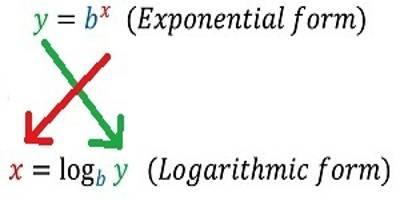Convert Exponentials and Logarithms

In convert Exponentials and Logarithms we will mainly discuss how to change the logarithm expression to Exponential expression and conversely from Exponential expression to logarithm expression. Logarithms are the “opposite” of exponentials, just as subtraction is the opposite of addition and division is the opposite of multiplication.

The logarithmic functions logb x and the exponential functions bx are inverse of each other, hence

y = logb x is equivalent to x = by

where b is the common base of the exponential and the logarithm.

The above equivalence helps in solving logarithmic and exponential functions and needs a deep understanding. Examples, of how the above relationship between the logarithm and exponential may be used to transform expressions, are presented below.

To discuss about convert Exponentials and Logarithms, we need to first recall logarithm and exponents.

The logarithm of any number to a given base is the index of the power to which the base must be raised in order to equal the given number. Thus, if aˣ = N, x is called the logarithm of N to the base a.For example:

1. Since 3⁴ = 81, the logarithm of 81 to base 3 is 4.
2. Since 10¹ = 10, 10² = 100, 10³ = 1000, …………

The natural number 1, 2, 3, …… are respectively the logarithms of 10, 100, 1000, …… to base 10.

The logarithm of N to base a is usually written as log₀ N, so that the same meaning is expressed by the two equations

ax = N; x = loga N

Examples on convert Exponentials and Logarithms

1. Convert the following exponential form to logarithmic form:

(i) 104 = 10000

Solution:

104 = 10000

⇒ log10 10000 = 4

(ii) 3-5 = x

Solution:

3-5 = x

⇒ log3 x = -5

(iii) (0.3)3 = 0.027

Solution:

(0.3)3 = 0.027

⇒ log0.3 0.027 = 3

1. Convert the following logarithmic form to exponential form:

(i) log3 81 = 4

Solution:

log3 81 = 4

⇒ 34 = 81, which is the required exponential form.

(ii) log8 32 = 5/3

Solution:

log8 32 = 5/3

⇒ 85/3 = 32

(iii) log10 0.1 = -1

Solution:

log10 0.1 = -1

⇒ 10-1 = 0.1.

1. By converting to exponential form, find the values of following:

(i) log2 16

Solution:

Let log2 16 = x

⇒ 2x = 16

⇒ 2x = 24

⇒ x = 4,

Therefore, log2 16 = 4.

(ii) log3 (1/3)

Solution:

Let log3 (1/3) = x

⇒ 3x = 1/3

⇒ 3x = 3-1

⇒ x = -1,

Therefore, log3 (1/3) = -1.

(iii) log5 0.008

Solution:

Let log5 0.008 = x

⇒ 5x = 0.008

⇒ 5x = 1/125

⇒ 5x = 5-3

⇒ x = -3,

Therefore, log5 0.008 = -3.

Information Source: